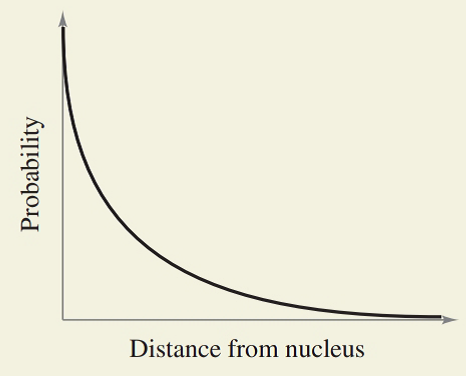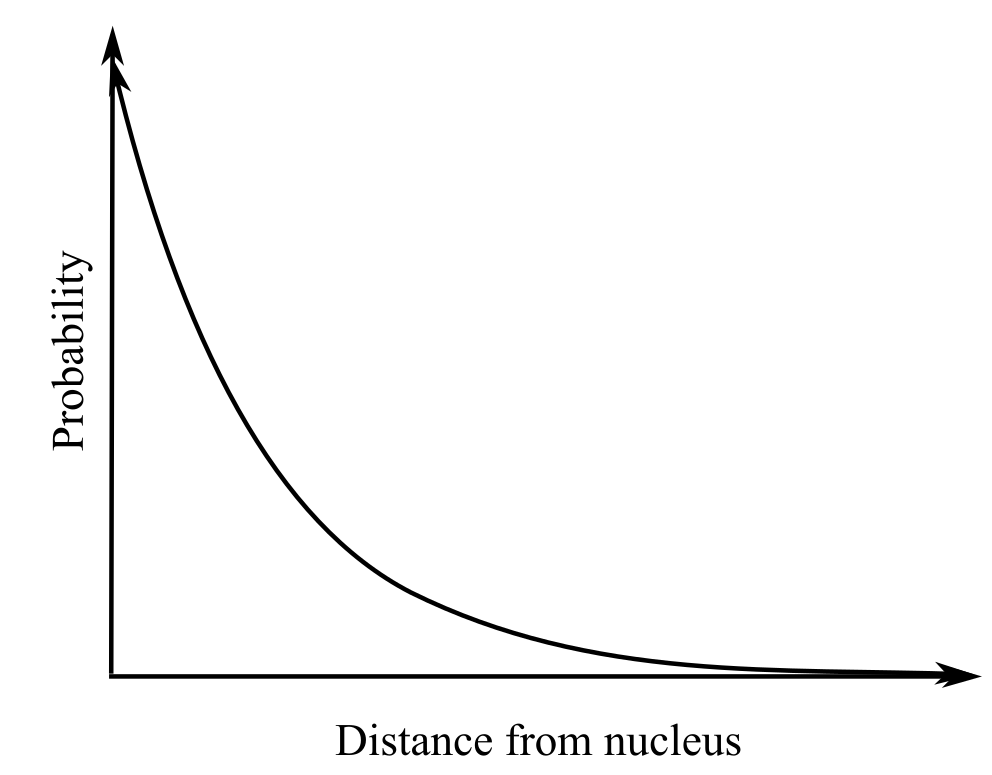Chapter 9.3, Problem 50E### Calculus: An Applied Approach (Min...

10th Edition
Ron Larson
ISBN: 9781305860919

#### Solutions

Chapter
Section### Calculus: An Applied Approach (Min...

10th Edition
Ron Larson
ISBN: 9781305860919
Textbook Problem
1 views

# HOW DO YOU SEE IT? In chemistry, the probability of finding an electron at a particular position is greatest close to the nucleus and drops off rapidly as the distance from the nucleus increases. The graph displays the probability of finding the electron at points along a line drawn from the nucleus outward in any direction for the hydrogen 1s orbital. Make a sketch of this graph, and add to your sketch an indication of where you think the median might be. (Source: Adapted from Zumdahl, Chemistry, Seventh Edition)To determine

To graph: The median in the provided graph as shown below:Explanation

Given Information:

The graph is shown below:

Graph:

Now consider the provided graph:

First label the interval on the y-axis and x-axis...

### Still sussing out bartleby?

Check out a sample textbook solution.

See a sample solution

#### The Solution to Your Study Problems

Bartleby provides explanations to thousands of textbook problems written by our experts, many with advanced degrees!

Get Started

#### Find more solutions based on key concepts